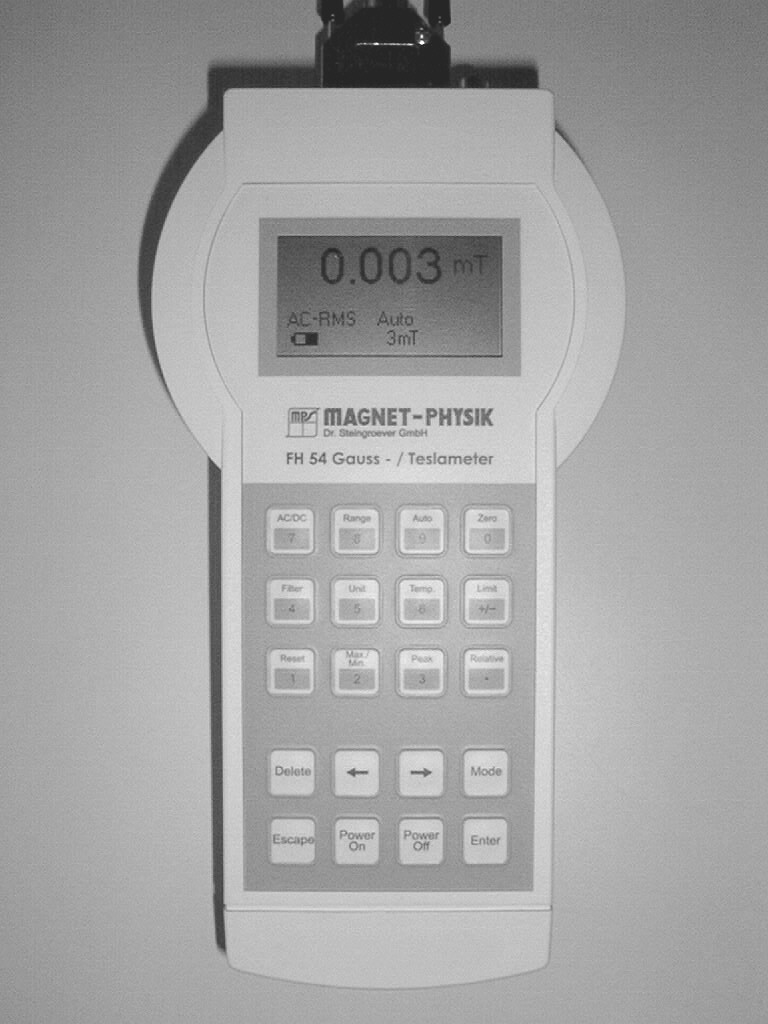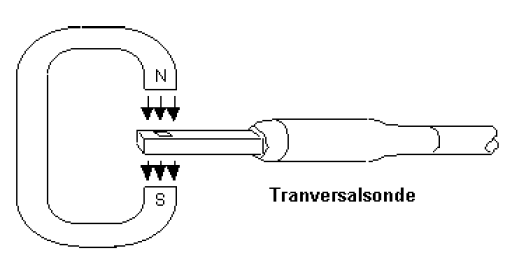Semiconductor Laboratory

## FH 54 MagnetometerThe FH 54 Magnetometer is a handheld measuring instrument for measuring the magnetic field strength H in Ampere per Meter (A/m) and the magnetic field (magnetic flux density) B in Tesla (T) or Gauss (G). It uses an analog output and a computer interface that allows data transfer and remote operation. The probe of the FH 54 is a Hall sensor that utilizes the Hall effect to measure the magnetic field.

The range in which the FH 54 magnetometer can measure the magnetic field depends on the probe that is mounted. In the Magnet Laboratory, a Magnet-Physik HS-TGB5-104020 probe is used, that has a range from 3 mT to 3 T. In this transversal probe, the Hall sensor is attached at the tip and along the broad side of the probe head. So to get an accurate reading of the magnetic field, you have to orient this broad side perpendicular to the direction of the magnetic field as shown in the figure below. An angle of 10° in any direction can produce an error of 1.5%. See the manual FH 54 Anleitung.pdf for more information on how a tilt effects the measurement. The probe has to be handled with uttermost care! The sensor of the probe is very brittle, be careful not to damage it.### Manual operation

Attach the Magnet-Physik HS-TGB5-104020 probe to the FH 54 (DA-15 connector), turn it on and let it warm up for 5 minutes. Although the FH 54 can be used battery powered, it is advised to use the power supply if you use it stationary. You can use the instrument to do AC (alternating, RMS or peak measurement) and DC (static) magnetic field measurements, if you measure the Bruker Magnet B-E 15 driven with the Heinzinger DC power supply you need to use the DC mode. A simple measurement can be performed by setting an appropriate range and the unit (Tesla) and carefully using the probe to measure the magnetic field of a permanent magnet (e.g. the ferromagnetic core of the Bruker Magnet B-E 15). The measurement is shown live at the display. Depending on the distance of the probe and its orientation relative to the permanent magnet, the measured value will change. To find the right orientation, the Max./Min. function can be used, press the button until the minimum and the maximum value are displayed. You can then change the orientation of the probe and use the orientation with the maximum magnetic field for further measurements. The Max./Min. values can be reset with the Reset button. It is important to note, that the FH 54 can, and should be zeroed, although it is not necessary to perform a zeroing before each measurement. But due to the zeroing being saved, you have to ensure, that the displayed value of the magnetic field is reasonable, e.g. if someone used the magnetometer and set the zero inside the magnet, your measured value will be of by this set zero. The Zeroing is performed with the Nullfeldkammer, that is provided with the FH 54. The exact procedure can be found in section 4.1 Inbetriebnahme of the manual.

### Communication with a computer

The FH 54 is connected to the computer with a serial (COM) port. There is currently no implementation of PyVISA in the library, so you don’t need a visa resource name but you have to know the name of the COM port that the FH 54 is connected to. In Windows this is usually along the lines of "COM1" and in Linux usually something like "dev/ tty1".

You can use the provided MagnetPhysik.py (recommended) library or connect directly using the serial communication library and the commands that are found in chapter 7 of the manual.

### Communication using the MagnetPhysik.py Python library

The MagnetPhysik.py library can be imported as shown in the structure example below. Structure of a Python script for the FH 54 Magnetometer:

Every command the FH 54 can execute is implemented in the MagnetPhysik.py library and can be looked up in the library. The important ones are described here.

`FH54()`
You have to create an instance of the FH54 class. In the code above, the instance was named `mm`.

`connect(self, port, baud_rate=19200, timeout=1)`
Function used to connect to the magnetometer over the serial interface, this function needs to be called before the other functions are available.
`port`: (string) The com port the magentometer is connected to. Under Windows usually something like "COM1", under Linux usually something like "dev/tty1".
`baud_rate`: (integer) The baud rate that is set at the magnetometer. You usually don’t have to set this.
`timeout`: (integer) Timeout for serial commands. You usually don't have to touch this.

```disconnect(self) Disconnect the serial port.```

``` set_mode_dc(self) Set the measurement mode to DC. You want to use this if you measure a static magnetic field or an DC driven electromagnet, and if you want to be sure that AC mode is not set. set_range(self, measurement_range) Set the range of the magnetometer like this:  set_range('3mT')  set_range('30mT')  set_range('300mT')  set_range('3T') It is advised to always choose a range for the measurement and don’t rely on the autorange function because the autorange of the FH 54 needs up to 2 seconds detect the range and also to not have deviations when the range changes. If you do not set the range or autorange in your script, it will use whatever range was used last with the FH 54, so be sure to set it. set_unit_Tesla(self) Set the displaying unit to Tesla. Usually you want to use this instead of the other possible units Gauss and Ampere_per_meter, you can always convert the units later if need be. If you don’t set the unit, it will choose the unit that was set last so always set the unit. read(self) Returns the current reading in the set unit. It will always return the value without the SI suffixes, e.g. a reading of 111 mT will be returned as ‘0.111’. The code below will use the Magnet-Physik FH 54 Magnetometer to measure the magnetic field of the Bruker Magnet B-E 15, powered by the Heinzinger PTN 125-40 power supply. from libs.Heinzinger import DigitalInterface from libs.MagnetPhysik import FH54 import matplotlib.pyplot as plt from datetime import datetime from time import sleep import numpy import csv """ Magnetic field measurement of the Bruker Magnet B-E 15, powered by the Heinzinger PTN 125-40 power supply, with the Magnet-Physik FH 54 Magnetometer. The sensor of the probe has to be mounted between the poles of the magnet (the orientation has to be normal for the measurement to be accurate). And the cooling of the magnet has to be turned on. """ """ Set the constants """ # set the ramp speed of the magnet power supply (Speed in A/s). SLOPE_SPEED <= 1 A/s SLOPE_SPEED = 0.25 # set the power supply current I_PS measure points (in Ampere) I_PS_START = 0 # # first measure point for I_PS (in Ampere) I_PS_END = 30 # # last measure point for I_PS (in Ampere) I_{S,max) = 30 A I_PS_POINTS = 121 # # number of measure points including I_PS_START and I_PS_END I_PS = numpy.linspace(I_PS_START, I_PS_END, num=I_PS_POINTS) """ If you want to do a hysteresis measurement (at least in the positive quadrant) you can use this instead of I_PS, it will append the values for the up measurement mirrored to itself to then perform a down measurement. I_PS_TEMP = numpy.linspace(I_PS_START, I_PS_END, num=I_PS_POINTS) I_PS = numpy.append(I_PS_TEMP, numpy.flipud(I_PS_TEMP)) """ # set the pause between setting the magnetic field and measuring the magnetic field in seconds PAUSE = 0.5 """ Configure the magnet power supply """ # connect to the magnet power supply ptn = DigitalInterface() ptn.connect("TCPIP0::129.27.158.19::7::SOCKET") # we want to ramp the current, starting with an initial current of 0 A ptn.ramp_current(0, slope=1) # when we ramp the current the magnet power supply is in constant current mode. # The voltage needs to be high enough so that the power supply doesn't switch to constant voltage mode ptn.set_voltage(100) """ Configure the magnetometer FH54 """ # connect to the FH54 magnetometer using the serial port, you have to use the COM port the magnetometer is plugged in mm = FH54() mm.connect("COM4") # set the measurement unit to Tesla mm.set_unit_Tesla() # set the range of the magnetometer mm.set_range(FH54.RANGE_3T) """ Saving the data """ # use date and time as a reference for saving the data DATEANDTIME = datetime.now().strftime("%Y-%m-%d_%Hh%Mm%Ss") # save the data as a csv # create a new file and define it as a csv. The name is: mf_meas_DATEANDTIME f = open("mf_meas_" + str(DATEANDTIME) + ".csv", "w") f_csv = csv.writer(f, delimiter=";", lineterminator='\n') # write headers into the csv file f_csv.writerow(["I_PS / A", "B / T"]) # define variables to store the data in B_measured = [] I_PS_measured = [] """ Do the measurement """ # measurement at every point in I_PS for set_current_ps in I_PS: # ramp up to the current in I_PS print("Ramp the current to " + str(set_current_ps) + " A") ptn.ramp_current(set_current_ps, slope=SLOPE_SPEED) sleep(PAUSE) current_ps = ptn.measure_current() mfield = mm.read() # save the measured values to display them B_measured.append(mfield) I_PS_measured.append(current_ps) # write the data in the csv file f_csv.writerow([current_ps, mfield]) # flush the csv file, so that it is saved f.flush() """ End the measurement """ # ramp down the magnetic field print("Shutting down the magnet power supply to 0 A (Speed 1 A/s)") ptn.ramp_current(0, slope=1) # for safety set the magnet power supply voltage to 0 ptn.set_voltage(0) # disconnect the magnet power supply ptn.disconnect() # disconnect from the magnetometer mm.disconnect() """ Show the data """ # plot the data fig_1 = plt.figure(1) plt.plot(I_PS_measured, B_measured) plt.xlabel("I_PS / A") plt.ylabel("B / T") # save the plot fig_1.savefig("mf_meas_" + str(DATEANDTIME)) plt.show() FH 54 Anleitung.pdf ```